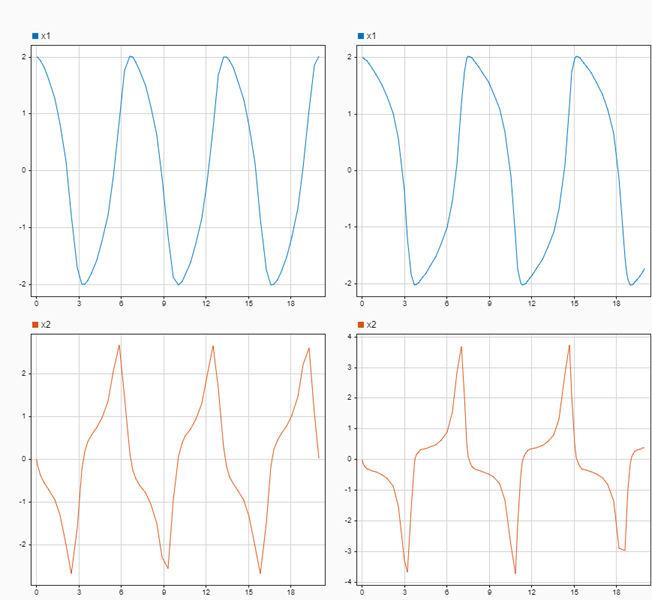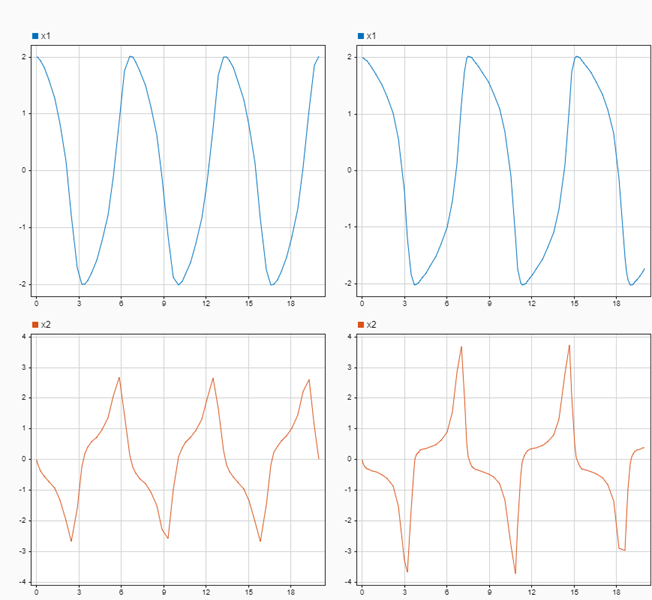Specify subplot limits for time plots in the Simulation Data Inspector

## Syntax

``Simulink.sdi.setSubplotLimits(r,c,Name,Value)``

## Description

example

````Simulink.sdi.setSubplotLimits(r,c,Name,Value)` configures the time and y-axis limits for the subplot at the location specified by `r` and `c` according to the values specified by one or more name-value pair arguments. You can specify any combination of a t-axis minimum, t-axis maximum, y-axis minimum, and y-axis maximum using name-value pair arguments. NoteLimits for the t-axis apply to all linked time plots in the Simulation Data Inspector. For more information, see Linked Subplots. ```

## Examples

collapse all

You can use the `Simulink.sdi.getSubplotLimits` and `Simulink.sdi.setSubplotLimits` functions to copy the axis limits from one subplot to another. For example, you can specify the same y-axis limits for two subplots that display the same signal from simulations that used different values of a model parameter. This example copies the y-axis settings from one subplot to another to analyze the effect of changing the value of `Mu` in the model `vdp`.

Create the data in the Simulation Data Inspector by simulating the model `vdp` twice. The first time, specify the value of `Mu` as `1`. For the second simulation, set the value of `Mu` to `2`. The model logs data for the signals `x1` and `x2`.

```open_system('ex_vdp'); set_param('ex_vdp/Mu','Gain','1') sim('ex_vdp'); set_param('ex_vdp/Mu','Gain','2'); sim('ex_vdp');```

Create plots in the Simulation Data Inspector to show the results from each simulation. Configure a `2x2` subplot layout so you can plot one signal on each plot, side by side.

`Simulink.sdi.setSubPlotLayout(2,2)`

Get the `Simulink.sdi.Signal` objects that correspond to each signal from each run, and plot one signal on each subplot. Plot the signals from the first simulation on the subplots in the first column and the signals from the second simulation on the subplots in the second column.

```runIDs = Simulink.sdi.getAllRunIDs; runID1 = runIDs(end-1); runID2 = runIDs(end); runMu1 = Simulink.sdi.getRun(runID1); runMu2 = Simulink.sdi.getRun(runID2); sig1 = getSignalByIndex(runMu1,1); sig2 = getSignalByIndex(runMu1,2); sig3 = getSignalByIndex(runMu2,1); sig4 = getSignalByIndex(runMu2,2); plotOnSubPlot(sig1,1,1,true); plotOnSubPlot(sig2,2,1,true); plotOnSubPlot(sig3,1,2,true); plotOnSubPlot(sig4,2,2,true);```

The signals in the first column of plots look similar to those in the second column. However, the y-axis limits for the plots of the `x2` signal are different.Use the `Simulink.sdi.getSubplotLimits` function to copy the limits used for the subplot with the `x2` signal from the second simulation.

`[~,~,ymin,ymax] = Simulink.sdi.getSubplotLimits(2,2);`

Use the `Simulink.sdi.setSubplotLimits` function to apply the same y-axis limits to the subplot with the `x2` signal from the first run.

`Simulink.sdi.setSubplotLimits(2,1,'yRange',[ymin,ymax]);`

With the updated y-axis limits, the difference in the `x2` signal is more apparent.## Input Arguments

collapse all

Subplot row index, specified as an integer between `1` and `8`, inclusive. Use the `r` and `c` inputs together to specify the location of the subplot for which you want to specify axis limits.

Example: `Simulink.sdi.setSubplotLimits(2,2,'yrange',[0,3])` configures y-axis limits for the time plot in the second row of the second column of the subplot layout in the Simulation Data Inspector.

Subplot column index, specified as an integer value between `1` and `8`, inclusive. Use the `r` and `c` inputs together to specify the location of the subplot for which you want to specify axis limits.

Example: `Simulink.sdi.setSubplotLimits(2,2,'yrange',[0,3])` configures y-axis limits for the time plot in the second row of the second column of the subplot layout in the Simulation Data Inspector.

### Name-Value Arguments

Specify optional comma-separated pairs of `Name,Value` arguments. `Name` is the argument name and `Value` is the corresponding value. `Name` must appear inside quotes. You can specify several name and value pair arguments in any order as `Name1,Value1,...,NameN,ValueN`.

Example: `Simulink.sdi.setSubplotLimits(2,2,'ymin',0,'ymax',3)` configures y-axis limits for the time plot in the second row of the second column of the subplot layout in the Simulation Data Inspector.

Simulation Data Inspector view, specified as `'Inspect'` or `'Compare'`. Specify the view with the subplot for which you want to specify limits. When you omit the `'View'` argument, the limits are applied to the specified subplot in the inspect view.

Example: `Simulink.sdi.setSubplotLimits(2,1,'View','Compare','yRange',[-2,2])` configures y-axis limits for the difference plot in the compare view of the Simulation Data Inspector.

Data Types: `char` | `string`

t-axis minimum, specified as a scalar.

You can specify limits for the t-axis using the `tMin` and `tMax` name-value arguments, using the `tRange` argument, or using the `AllRange` argument. When you specify the `tMin` name-value pair argument, do not specify the `tRange` or `AllRange` arguments.

Data Types: `double`

t-axis maximum, specified as a scalar.

You can specify limits for the t-axis using the `tMin` and `tMax` name-value arguments, the `tRange` argument, or the `AllRange` argument. When you specify the `tMax` name-value argument, do not specify the `tRange` or `AllRange` arguments.

Data Types: `double`

y-axis minimum, specified as a scalar.

You can specify limits for the y-axis using the `yMin` and `yMax` name-value arguments, the `yRange` argument, or the `AllRange` argument. When you specify the `yMin` name-value argument, do not specify the `yRange` or `AllRange` arguments.

Data Types: `double`

y-axis maximum, specified as a scalar.

You can specify limits for the y-axis using the `yMin` and `yMax` name-value arguments, the `yRange` argument, or the `AllRange` argument. When you specify the `yMax` name-value argument, do not specify the `yRange` or `AllRange` arguments.

Data Types: `double`

t-axis range, specified as a `1`-by-`2` vector that contains scalar numeric values.

You can specify limits for the t-axis using the `tMin` and `tMax` name-value arguments, the `tRange` argument, or the `AllRange` argument. When you specify the `tRange` name-value argument, do not specify the `tMin`, `tMax`, or `AllRange` arguments.

Example: `Simulink.sdi.setSubplotLimits(1,1,'tRange',[-1,20])` configures the t-axis range for the subplot in the first row and first column of the Simulation Data Inspector subplot layout.

Data Types: `double`

y-axis range, specified as a `1`-by-`2` vector that contains scalar numeric values.

You can specify limits for the y-axis using the `yMin` and `yMax` name-value arguments, the `yRange` argument, or the `AllRange` argument. When you specify the `yRange` name-value argument, do not specify the `yMin`, `yMax`, or `AllRange` arguments.

Example: `Simulink.sdi.setSubplotLimits(1,1,'yRange',[-5,5])` configures the y-axis range for the subplot in the first row and first column of the Simulation Data Inspector subplot layout.

Data Types: `double`

Ranges for t-axis and y-axis, specified as a `1`-by-`4` vector that contains scalar numeric values. The values specified in the vector are mapped to the t- and y-axis limits in this order: [`tMin`,`tMax`,`yMin`,`yMax`].

When you specify the `AllRange` name-value argument, do not specify the `tMin`, `tMax`, `yMin`, `yMax`, `tRange`, or `yRange` arguments.

Example: `Simulink.sdi.setSubplotLimits(1,1,'AllRange',[-1,20,-5,5])` configures the t-axis range and y-axis range for the subplot in the first row and first column of the Simulation Data Inspector subplot layout.

Data Types: `double`

Introduced in R2021a